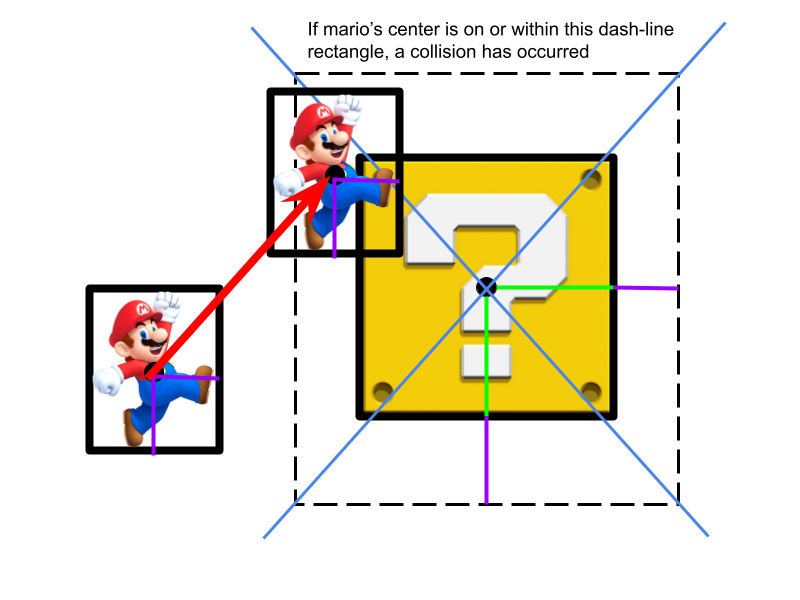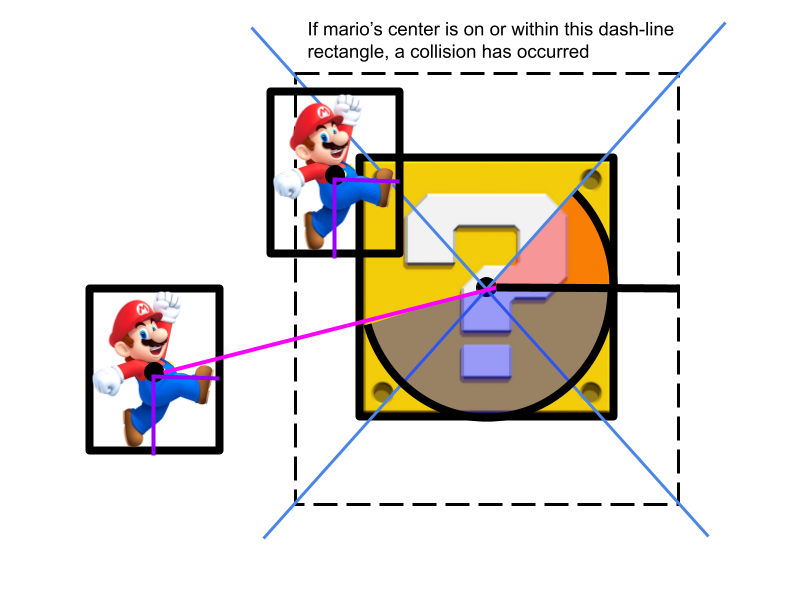# Collisions

Collisions are one of the most important and fundamental aspects of game development. Based on when and how certain things collide, events occur to make the game dynamic and fun to play. In this lesson, we will go over how to implement collisions in our demo.

### Theory Behind Collisions

Collisions in any game are all about the type of the collision and its subsequent event. For example, if a player jumps and there is a block above him, the upward collision (type) triggers a change in the player’s trajectory (event). This means it is important that we reliably get the type of collision so that we can fire the right event. Below shows how we do this.### Checking if there is a Collision

First of all, we have to check if a collision has happened at all. To do this, we can check the horizontal and vertical distance between the center of mario and the box, and if both of them are shorter than a critical distance (which we will define later) at the same time, then a collision has occurred.

Let’s first look at just getting the distance between the centers horizontally. How do we get a distance in the first place? We can simply subtract the position of one point by the position of the other, which gives us the difference/displacement between their positions. This might give us a negative value though, so we just put the result in an absolute value function (abs()) to get the raw distance. We can use this same concept to find the vertical distance.

``````abs(player[distFromStart]-component[distFromStart])
``````

Now that we have our distances, we have to find our “critical distances” for the horizontal distance and the vertical distance. This is relatively simple. If we go back to just looking at horizontal distance, we can find our critical distance by adding half of mario’s width to half of the box’s width. This makes sense, since this would be the minimum horizontal distance that could be in between the center of the box and mario. So, if we apply this to vertical distance (using half-heights instead), we can determine if there is a collision or not by seeing if both critical distances have been “breached” at the same time.

``````if (
abs(player[distFromStart]-component[distFromStart]) <= (player[run]+component[run])/2
&&
abs(player[distFromBottom]-component[distFromBottom]) <=(player[rise]+component[rise])/2
)
``````

### Determining the Type of Collision

Ok, so we can now figure out if there is a collision. Now onto the more complex part: how do we determine the type of the collision? Did Mario hit his head? Did he land on a block? Did he run into a block? Now you will figure out how to extract this information using code and a little bit of math.First, we need to get context. Where, relative to the block, was Mario right before the collision? The first thing we need to do is store Mario’s coordinates right before the collision. We do this in the PVector variable prevPoint.

``````prevPoint = new PVector(player[distFromStart],player[distFromBottom]);
``````

Now that we have this stored, we can determine how Mario hit the block. Take a look at the picture above. Outlined are 4 sections that split up the space surrounding the block, and Mario’s prevPoint belongs in the left section. If you draw a line from this point to where mario is now, you will see that line intersects with the left side of the collision rectangle, outlined with dash-line. In other words, in order for Mario to have collided with the box, he must have first passed through the left part of the collision rectangle. This means he must have collided with the box on the left side, which gives us our type of collision.

In fact, if Mario’s prevPoint were to have originated from the left section of the surrounding area, it works out so that any collision with the box would be a left-sided collision from the box, or a right sided collision relative to mario. So all we need to do is define each section, as well as in which section the prevPoint resides in.To determine which quadrant Mario’s prevPoint was, we can define the quadrants by taking a “critical angle” using atan2(), which helps us define the quadrants themselves. Then, we will find the angle produced by the line joining prevPoint and the center of the box. Using some if statements, we can figure out which angles correspond to which quadrants, and thus we can find the nature of the collision!